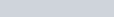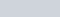In this video, you are introduced to the method of iteration in order to solve an equation.

To demonstrate this method, the following example is used.

Example:

1. Find one root of the equationby iteration taking the first approximationgiving the answer to 2 decimal places.

## How it works:

In this video, you are shown how iteration converges or diverges away from a root by considering the intersection of the graph y=x and y=f(x)